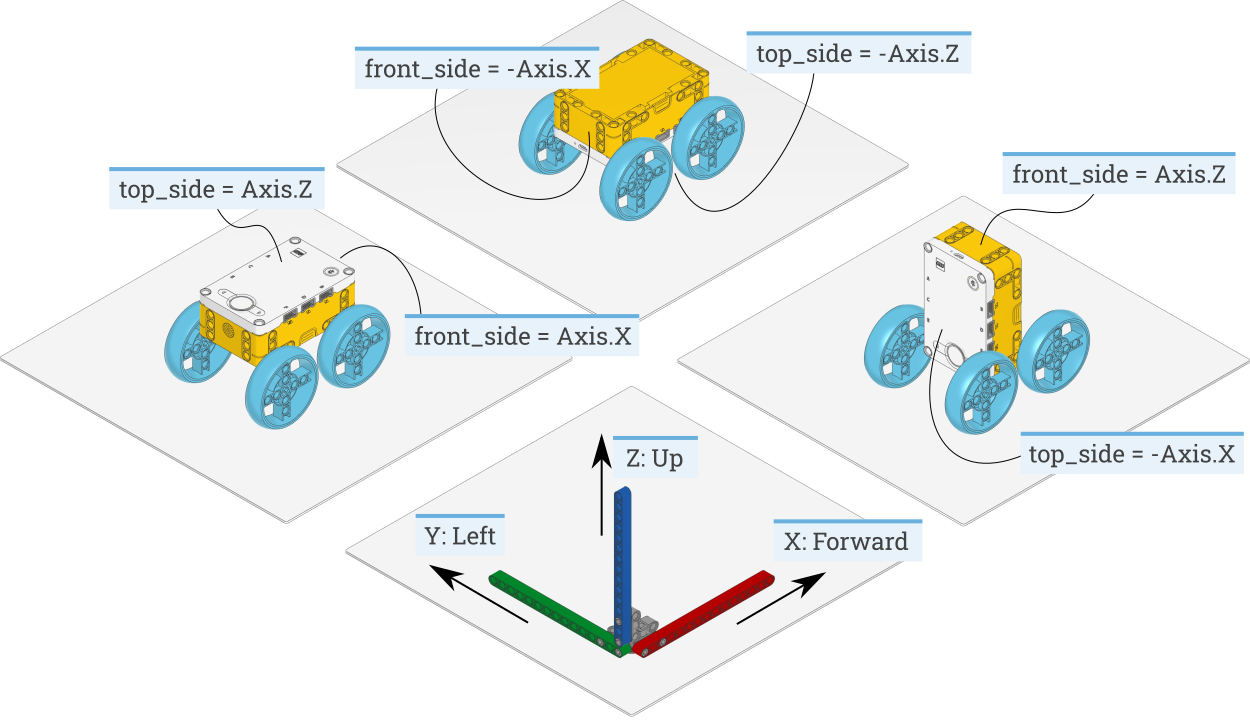# `geometry` – Geometry and algebra¶

class Matrix(rows)

Mathematical representation of a matrix. It supports addition (`A + B`), subtraction (`A - B`), and matrix multiplication (`A * B`) for matrices of compatible size.

It also supports scalar multiplication (`c * A` or `A * c`) and scalar division (`A / c`).

A `Matrix` object is immutable.

Parameters

rows (list) – List of rows. Each row is itself a list of numbers.

T

Returns a new `Matrix` that is the transpose of the original.

shape

Returns a tuple (`m`, `n`), where `m` is the number of rows and `n` is the number of columns.

vector(x, y)
vector(x, y, z)

Convenience function to create a `Matrix` with the shape (`2`, `1`) or (`3`, `1`).

Parameters
• x (float) – x-coordinate of the vector.

• y (float) – y-coordinate of the vector.

• z (float) – z-coordinate of the vector (optional).

Returns

A matrix with the shape of a column vector.

class Axis

Unit axes of a coordinate system.

X = vector(1, 0, 0)
Y = vector(0, 1, 0)
Z = vector(0, 0, 1)

## Reference frames¶

The Pybricks module and this documentation use the following conventions:

• X: Positive means forward. Negative means backward.

• Y: Positive means to the left. Negative means to the right.

• Z: Positive means upward. Negative means downward.

To make sure that all hub measurements (such as acceleration) have the correct value and sign, you can specify how the hub is mounted in your creation. This adjust the measurements so that it is easy to see how your robot is moving, rather than how the hub is moving.

For example, the hub may be mounted upside down in your design. If you configure the settings as shown in Figure 4, the hub measurements will be adjusted accordingly. This way, a positive acceleration value in the X direction means that your robot accelerates forward, even though the hub accelerates backward.Figure 4 How to configure the top_side and front_side settings for three different robot designs. The same technique can be applied to other hubs and other creations, by noting which way the top and front Side of the hub are pointing. The example on the left is the default configuration.¶